### 朴素贝叶斯算法理论

【[5分钟学算法] #02 朴素贝叶斯 写作业还得看小明-哔哩哔哩】 https://b23.tv/tjH0mpe

### 任务目标

``````types=["新闻","体育","汽车","房产","旅游","教育","时尚","科技","财经","娱乐"]
``````

### 准备数据

dataset.xlsx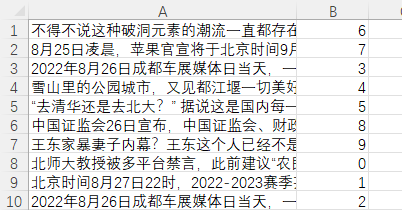``````import openpyxl

sheet=wb.get_sheet_by_name(sheet_name)
datas=[]
for row in sheet.iter_rows():
data=[col.value for col in row]
datas.append(data)
return datas
``````

### 文本分词

``````import jieda
import jieba.analyse

def getStops(fpath="stops.txt"):#加载停词表
f=open(fpath,'r',encoding="utf-8")
f.close()
stops=page.split("\n")
return stops

def tokenize(content):#通过jieba分词，然后用停词表剔除停词，最后去重返回
keywords=jieba.cut(content)
stops=getStops()
newkeywords=[]
for kw in keywords:
if kw not in stops:
newkeywords.append(kw)
return set(newkeywords)
``````

stops.txt

``````--
?
“
”

``````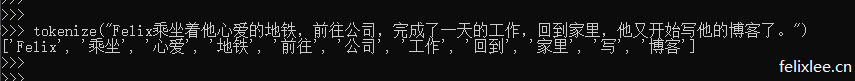### 计算一系列单词在各个类别出现的频次

``````from collections import defaultdict

#返回一系列单词分别出现在各个类别的次数 有几个类别，value就有几个元素，比如是10个分类就是一个10个元素的list，初始值都是0，用于储存该关键词在不同类别出现的次数。
def count_words(training_set,types):
counts=defaultdict(lambda:[0 for i in range(len(types))])
for content,type_index in training_set:
for keyword in tokenize(content):
counts[keyword][type_index] += 1  #counts[keyword]是单词出现在类别0中的数量 counts[keyword] 则是该单词在类别1中出现的次数，以此类推
return counts
``````

defaultdict是python的一个工厂函数，可以在传入某个不存在的key的时候，返回一个默认的值，故名为defaultdict，它接受一个函数作为返回的默认值。

``````import random

random.shuffle(dataset)#打乱数据集
#拆分训练集与测试集
training_set=dataset[:70]#取打乱后的数据集的前70条作为训练集
test_set=dataset[70:]#取后30条作为测试集

word_counts=count_words(training_set,types)#计算词频
``````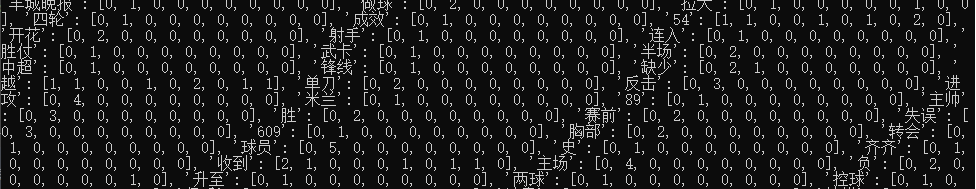### 计算一系列单词在各个类别出现的频率

``````def word_probabilities(counts,type_total_counts,types,k=0.5):
word_probs=[]
for w,w_all_counts in counts.items():
word_prob=[w]
word_prob_list=[]
for i in range(len(w_all_counts)):
w_onetype_counts=w_all_counts[i]
onetype_total_counts=type_total_counts[i]
word_prob_list.append((w_onetype_counts+k)/(onetype_total_counts+len(types)*k))
word_prob.append(word_prob_list)
word_probs.append(word_prob)

return word_probs
``````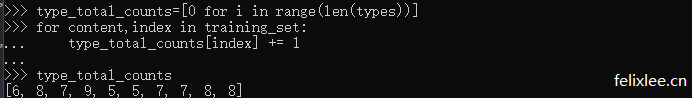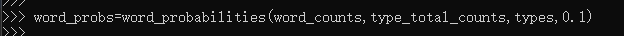word_probabilities函数返回的结果格式为

``````[
["单词1",["单词1在分类1的概率","单词1在分类2的概率","单词1在分类3的概率",...,"单词1在分类10的概率"]],
["单词2",["单词2在分类1的概率","单词2在分类2的概率","单词2在分类3的概率",...,"单词2在分类10的概率"]],
...
["单词n",["单词n在分类1的概率","单词n在分类2的概率","单词n在分类3的概率",...,"单词n在分类10的概率"]]
]
``````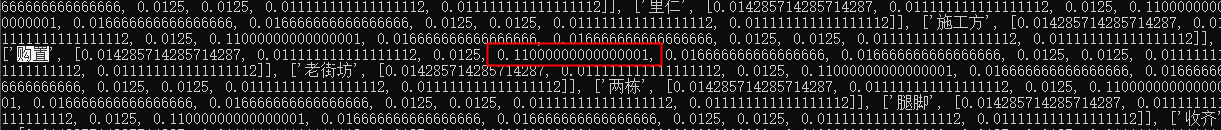### 根据训练的概率对新内容进行预测

``````import math

#根据训练的概率，返回新文本属于各类别的概率
def word_probability(word_probs,content,types):
content_words=tokenize(content)
log_probs_type=[0.0 for i in range(len(types))]

for word,probs in word_probs:
if word in content:#如果文本包含训练数据中的某个词就在（文本属于某个类别的概率）上加上（这个词在某个类别的概率）
for i in range(len(probs)):
prob=probs[i]
log_probs_type[i] += math.log(prob)
else:#如果文本不包含训练数据中的某个词就在（文本属于某个类别的概率）上加上（1 减去 这个词在某个类别的概率）
for i in range(len(probs)):
prob=probs[i]
log_probs_type[i] += math.log(1-prob)

return log_probs_type
``````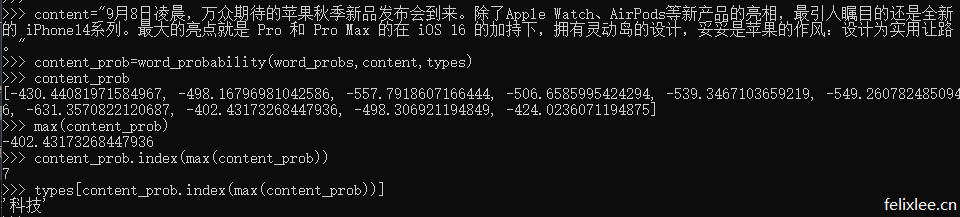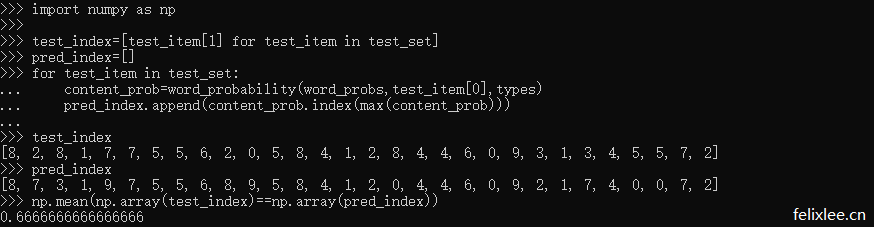### 封装打包

``````class NaiveBayesClassifier:
def __init__(self,types,k=0.5):#初始化
self.k=k
self.word_probs=[]
self.types=types

def train(self,training_set):#训练方法
type_total_counts=[0 for i in range(len(self.types))]
for content,index in training_set:
type_total_counts[index] += 1
word_counts=count_words(training_set,self.types)
self.word_probs=word_probabilities(word_counts,type_total_counts,self.types,self.k)

def classify(self,content):#获得属于各类别的概率
return word_probability(self.word_probs,content,self.types)

def gettype(self,content):#获得预测类别
classify_probs=self.classify(content)
max_prob=max(classify_probs)
max_prob_index=classify_probs.index(max_prob)
return self.types[max_prob_index]

def score(self,test_set):#传入测试集
test_index=[test_item for test_item in test_set]
pred_index=[]
for test_item in test_set:
content_prob=word_probability(self.word_probs,test_item,self.types)
pred_index.append(content_prob.index(max(content_prob)))

return np.mean(np.array(test_index)==np.array(pred_index))
``````

``````>>> types=["新闻","体育","汽车","房产","旅游","教育","时尚","科技","财经","娱乐"]
>>> random.shuffle(dataset)
>>> training_set=dataset[:70]
>>> test_set=dataset[70:]

>>> nbc=NaiveBayesClassifier(types)
>>> nbc.train(training_set)
>>> content="""北京时间9月14日消息，2022赛季中超联赛第18轮联赛继续进行，北京国安在日照主场迎来了副班长河北队的挑战。上半场张稀哲点射，陈运华扳平，王子铭建功，下半场张玉宁锁定胜局，最终北京国安3-1击败河北队，新帅斯坦利上任后取得首胜。国安在换帅 之后，上轮联赛2-2战平了浙江队，尽管在技战术层面并没有过多的变动，但是球员们的精气神却与以往不同，斗志更强了，本轮对阵垫 底的河北队，两队实力相差明显，国安新帅斯坦利大概率将会在本场比赛展现出更多的自己带队的风格，如果取胜，那么御林军也将会在积分榜上继续向前追赶。相关阅读-媒体人：国足选帅别急着上马 不想明白谁上也白废比赛开始后第4分钟，高天意在中路寻求突破，随 后左脚远射被鲍亚雄轻松抱住；第6分钟，张稀哲开出左侧任意球，柏杨禁区内争顶头球攻门高出横梁；第9分钟，高华泽禁区右侧过掉姜祥佑之后小角度抽射被柏杨挡出；第15分钟，于大宝中场输送直塞，张玉宁甩开防守不停球直接爆射被鲍亚雄扑出底线；第16分钟，张稀哲开出角球被解围，随后他再次传中，于大宝后点头球回做，王子铭将球拨进球门，不过经过VAR回看，助攻的于大宝在张稀哲传球时越 位，进球无效；"""
>>> nbc.classify(content)
[-1100.7245522663443, -1007.8436308839352, -1230.5955078582558, -1131.7388604926687, -1168.1510738024772, -1233.9717449797113, -1201.7283979422293, -1157.891144823666, -1119.596931376088, -1190.116854852187]
>>> nbc.gettype(content)
"体育"
``````

### 其他

btw，这本《数据分析入门》还是很适合新入门机器学习的朋友的，不是上来就告诉你怎么掉包，而是将实现原理告诉你，并将实现代码附上。之前看过的《Python机器学习基础教程》，其实也不错，k近邻这一章用莺尾花的例子将这个方法讲得很透彻，不过越到后面，对于细节描述会更少，对于像我们这种统计学基础较少的小白来说还是不太友好的，更多告诉你如何调参数，不太利于理解。不过你原本就有不错的统计学、概率论基础，看这本书应该也是个不错的选择。另外本人在机器学习方面仍处于摸索阶段，如有不正确之处还望海涵，若能指出问题更是感激不尽。• #### 最新随笔

• 最近两周也没咋出去玩，主要也是觉得没啥好玩的（笑哭）。看完布莱恩阿瑟的《复杂经济学》后，里面那个酒吧问题勾起我的兴趣，最近空了就花了些时间实现个python版本，顺便搞了篇博文，很享受这种新知识能和已有知识碰撞的感觉。（配张前段时间拍的图片，梧桐山门口前面那条路，挺漂亮的）
• 盐田港夜景
• 为啥这猫总喜欢喝杯子里的水
• 确实开始冷了，在树林里至少要比人类聚集区低个几度，进出入口就能很明显感觉到。看看深圳水库的风景，貌似后面的视野更开阔。
• 给随笔加了多图的功能，传一传周末拍的风景图，漫无目的的逛也挺好玩的。
• 逻辑自洽是一套体系的根基，最根本的因素，最吸引人的地方
• 这个水库风景好美，而且没啥人，只有树叶和鸟叫声，天气也好，很凉爽。水面波光粼粼，看久了有种做梦的感觉。
• 甘坑古镇晚上好看，跟阿仙来的，但人有点多，不过人姑且也算景之一，为啥？烟火气算吧。